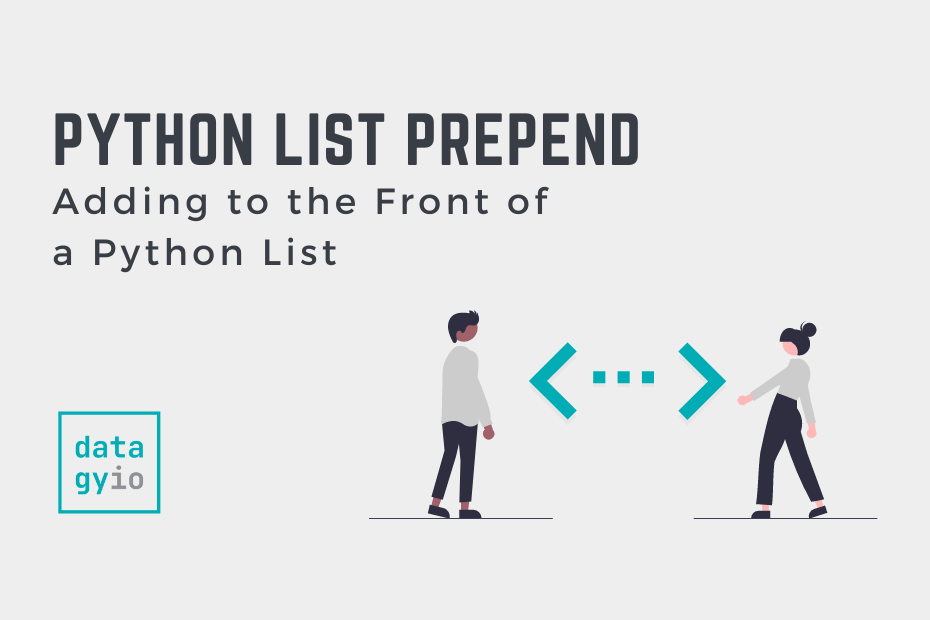# Prepend to a Python a List (Append at beginning)In this tutorial, you’ll learn how to use Python to prepend a list. While Python has a method to append values to the end of a list, there is no prepend method. By the end of this tutorial, you’ll have learned how to use the `.insert()` method and how to concatenate two lists to prepend.

You’ll also learn how to use the `deque` object to insert values at the front of a list. In many cases, this is the preferred method, as it’s significantly more memory efficient than other methods.

## The Problem with Prepending a Python List

Python lists are a mutable container data type, meaning that they can be altered. Because of this, it can be tempting to add an item to a list. However, depending on the size of your list, this process can be immensely memory intensive.

The reason for this is that there is no “room” at the beginning of the list. When you add an item to the front of a list, Python needs to shift all other items forward. Later in this tutorial, you’ll learn about the `deque` data structure, which represents a double-ended queue. In these deque objects, items can be inserted freely at the beginning or end.

## Using Insert to Prepend to a Python List

An intuitive way to add an item to the front of a Python list is to use the insert method. The `.insert()` method takes two parameters:

1. The index position to insert the item in, and

Let’s take a look at an example:

``````# Using .insert() to prepend to a Python list
words = ['welcome', 'to', 'datagy']
words.insert(0, 'hello')

print(words)

# Returns: ['hello', 'welcome', 'to', 'datagy']``````

The operation takes place in-place, meaning that a new object doesn’t need to be created. In the following example, you’ll learn how to use the `+` operator to prepend an item to a list.

## Using the + Operator to Prepend to a Python List

The Python `+` operator is an incredibly versatile operator. When combined with two lists, the two lists are added together, in the order in which they appear. This means that when we want to prepend an item (or multiple items), we can create a list containing this item.

We then only need to apply the `+` operator between the two lists to combine them. Let’s take a look at another example:

``````# Using + to prepend an item to a list
words = ['welcome', 'to', 'datagy']
prefix = ['hello']

words = prefix + words
print(words)

# Returns: ['hello', 'welcome', 'to', 'datagy']``````

Similarly, we can use the augmented assignment operator to prepend an item to a list. The difference here is that we need to reassign to the prefix, not the other way around. This is demonstrated below:

``````# Using the augmented assignment operator to prepend an item to a list
words = ['welcome', 'to', 'datagy']
prefix = ['hello']

prefix += words
print(prefix)

# Returns: ['hello', 'welcome', 'to', 'datagy']``````

In the next section, you’ll learn how to use list slicing to prepend to a Python list.

## Using List Slicing to Prepend to a Python List

This method can feel a bit awkward, but it can also be a useful way to assign an item to the front of a list. We assign a list with a single value to the slice of `[:0]` of another list. This forces the item to be added before the 0th item.

Let’s take a look at an example:

``````# Using List Slicing to prepend to a Python List
words = ['welcome', 'to', 'datagy']
words[:0] = ['hello']

print(words)

# Returns: ['hello', 'welcome', 'to', 'datagy']``````

In the final section, you’ll learn the most memory-efficient way to add items to the front of a Python list.

## Using Deque to Prepend to a Python List

Part of the incredibly versatile `collections` library is the `deque` class. This class represents a double-ended queue, which represent stacks or queues from other languages. The benefit of this is that the class allows you to prepend items to the left, without the memory implications of shifting all values.

Let’s take a look at an example:

``````# Using deque to prepend to a list in Python
from collections import deque
words = deque(['welcome', 'to', 'datagy'])
words.appendleft('hello')

print(words)

# Returns: ['hello', 'welcome', 'to', 'datagy']``````

The benefit of this approach is noticeable when working with large lists. With smaller lists, you may not notice a benefit, but it’s a good idea to keep performance in mind.

## Conclusion

In this tutorial, you learned how to prepend to a Python list. You first learned why prepending to a list can be a troublesome idea. Then, you learned how to use three different list methods, including `.insert()`, the `+` operator, and list indexing to prepend to a list. Finally, you learned how to use the collections `deque` object to prepend to a list in a memory-efficient manner.